top of page
Search
• Stu WØSTU

# Complex Impedance Part 3: Putting It All Together

In Part 1 of this series, Just a Passing Phase, we defined the sine waveform of the most common AC signals, illustrated how AC voltage and current each vary as a sine waveform in AC circuits, and we examined the concept of phase angles in describing the alignment (or lack of it) between a voltage sine wave and a current sine wave.

In Part 2, Reacting Nicely, we examined a bit of the dynamics of voltage and current within capacitors and inductors to get a sense for just how these components impose phase angles between voltage and current sine waves. We described the concept of reactance and illustrated the calculations of capacitive and inductive reactances.

Returning to the big picture points laid out in Part 1 and Part 2:

1. Voltage and current applied to AC circuits are each represented by smoothly changing sine waveforms of equal frequency, depicting the regular reversals of direction and smoothly changing magnitudes of each.

2. The applied voltage and current sine waves often get out of step with one another so the two representations no longer oscillate together, as if one sine wave is shifted ahead or behind the other in time, or phase.

3. The amount of deviation between the voltage and current sine wave signals in a circuit is described by a phase angle between the two signals, in units of degrees.

4. Phase angle shifts between voltage and current are imposed by a type of opposition to current flow called reactance in AC circuit components, specifically inductive reactance and capacitive reactance, measured in units of ohms.

5. Inductive and capacitive reactances combine in a complex way with resistance in a circuit to determine the overall impedance of the circuit.

6. Complex impedance is described with both a magnitude in ohms and a phase angle in degrees, and there are two primary shorthand methods of representing complex impedance in writing (rectangular and polar forms).

7. Impedance magnitude and phase angle impact the behavior of AC circuits, particularly with respect to power transfer and resonance, as in RF antenna circuits, oscillator circuits, matching networks, power supply circuits, and many others.

We covered points 1 through 3 in Part 1. In Part 2 we focused on point 4. We will now complete this series with elaboration of points 5, 6, and 7. These really put it all together!

Complex Impedance: You may recall that impedance (Z) is defined as the opposition to the flow of current in an AC circuit. Impedance combines the effects of simple resistance with reactance due to capacitive and inductive components in the circuit. However, the relationship among resistance, capacitive reactance, and inductive reactance is more complex than simple addition of each factor. Let’s consider the interactions among resistance and reactances in more detail on the way to understanding and computing the impedance of a circuit.

First, resistance in a circuit with capacitors and/or inductors will affect the phase angle between voltage and current. The “pure” cases of only capacitance or only inductance that we considered in Part 2 are only ideal models that provide a perfect 90-degree phase angle between voltage and current sine waves. All real circuits will have some resistance due to wires and components, and often will also have component resistors, all of which shift the phase angle when combined with capacitors and/or inductors. So, the phase difference between voltage and current can be angles less than 90 degrees, and the precise phase angle depends on the relative values of resistance and reactance in the AC circuit.

Resistance affects the phase angle between voltage and current in AC circuits containing capacitive and inductive components.

Second, resistance and reactance combine as vectors and not via simple addition. We will examine “vector addition” in a moment using the graphs below.

Third, inductive reactance and capacitive reactance have opposite effects on phase angle. Remember, an inductive reactance makes the voltage lead the current, while a capacitive reactance makes the current lead the voltage. When inductive and capacitive components are combined in a series circuit these opposing reactances negate one another, in part or in whole, thereby determining the resultant reactance of the series circuit and impacting the overall impedance of the circuit.

Inductive reactance and capacitive reactance in a series AC circuit offset one another.

Series Circuit Impedance: Currently, we will consider only series circuit cases. Parallel circuits require a bit of a twist on the series case and we will consider them later in this article. Let’s take an example series circuit problem derived from the Extra Class question pool and solve it using a vector graph of the circuit, with explanation along the way.

Q. What is the impedance of a circuit consisting of a 400-Ω resistor in series with an inductor that has 300-Ω of reactance?

As we noted above, resistance and reactance add as vectors. We can depict this graphically using a common rectangular coordinate plane and plotting a vector for resistance and reactance. By standard convention, we will plot the resistance value along the X axis (horizontal axis), and we will plot reactance along the Y (vertical axis).

In this first example we have 400-Ω resistance, so we will draw a vector from the origin (0, 0) along the horizontal resistance axis to 400, or the coordinate plane position (400, 0).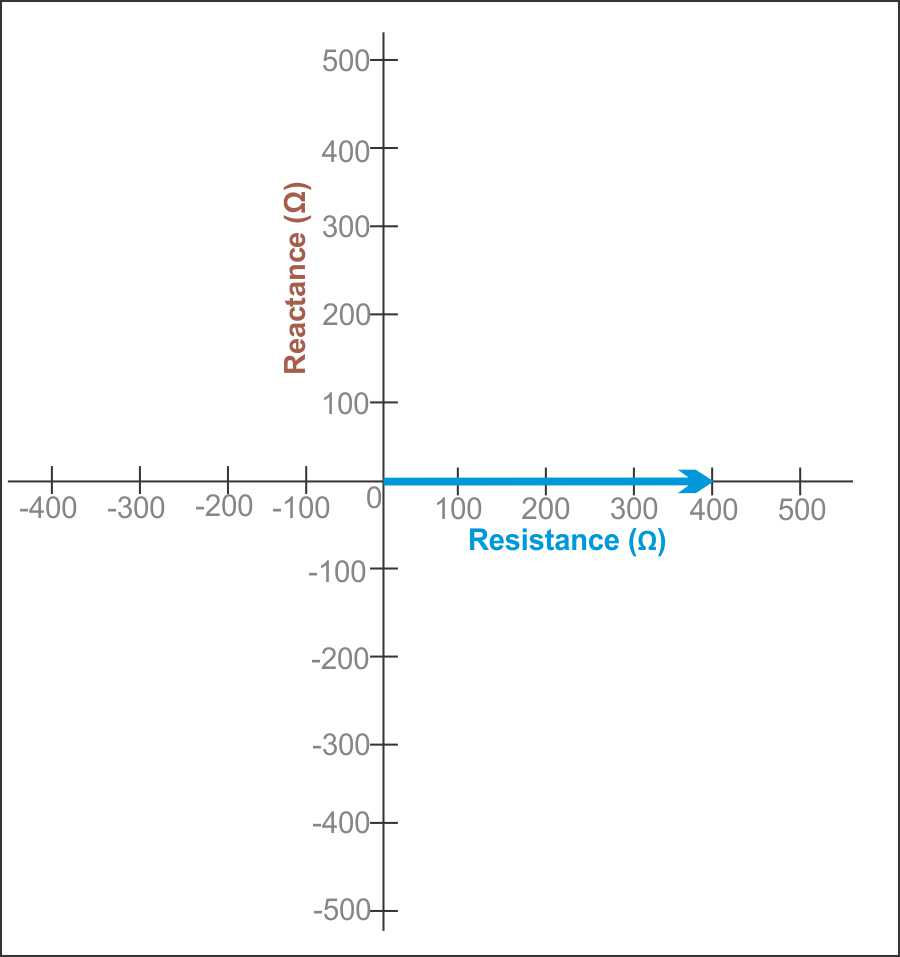Resistance value plotted as vector.

Next, we must plot a 300-Ω inductive reactance. In order to add these two vectors we must start this inductive reactance vector at the head of the already-plotted resistance vector, or at the point (400, 0). By standard convention, all inductive reactance is plotted in the positive vertical axis, or “upward” in the coordinate plane.

Since the inductive reactance is 300-Ω, we draw a vector straight up from the (400, 0) position to a position 300 units above. The point we have reached is the coordinate (400, 300).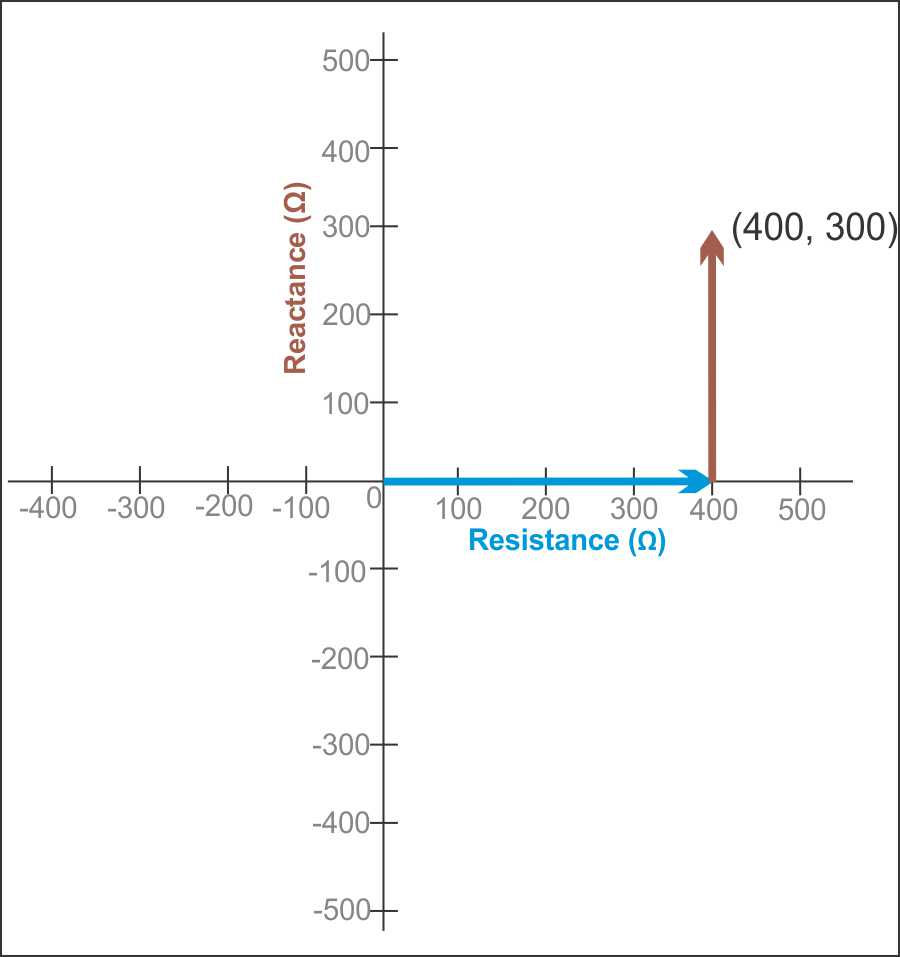Inductive reactance vector added to resistance vector.

Now, we complete the vector diagram by connecting the origin with the vector resultant position, or a vector extending from (0, 0) to (400, 300).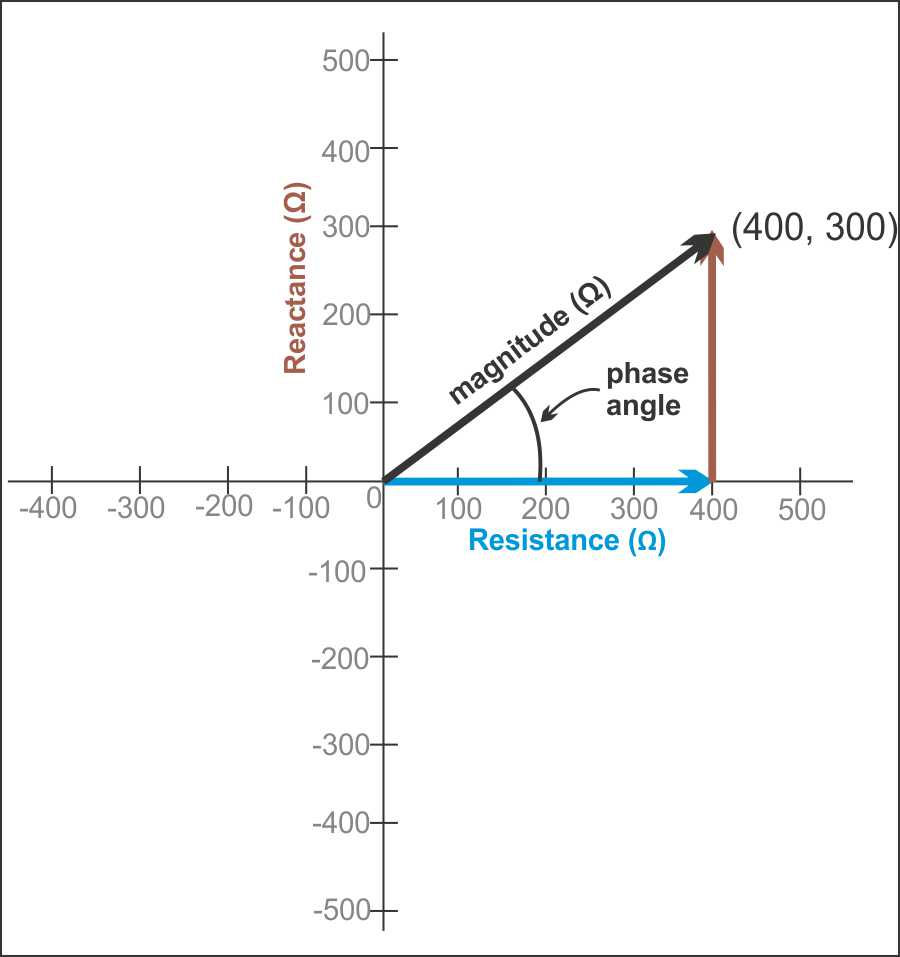Resultant impedance vector depicts magnitude and phase angle.

In the parlance of rectangular coordinates like this, we use the following shorthand to represent this impedance picture:

Z = 400 + j 300 Ω

To express complex impedance (Z) in rectangular form first state the value of the resistance (400) and tack on value of the reactance (+300) with the lowercase letter ‘j’ preceding it, as shown above. Consider the j as a special designator to indicate that the value following it is reactance.

The resultant vector in the rectangular coordinate system picture represents the series circuit’s complex impedance. We can express it in the rectangular shorthand above or we may convert to polar form notation. Notice that the resultant vector has a length that represents the magnitude of the impedance in ohms and it has an angle as measured counterclockwise from the horizontal resistance axis that represents the phase angle between voltage and current. In order to properly state the complex impedance of the circuit in polar form we must compute both the magnitude (length) and the angle.

Call upon some middle school math with the Pythagorean Theorem that works for all right triangles like the one resulting from our vector plots. The side opposite the right angle (90-degree angle) is always the hypotenuse, and it is always the longest side of the right triangle. This is the side depicting the magnitude of impedance. We will call that side ‘C’. The other two sides we will dub ‘A’ and ‘B’. The theorem states, as I’m sure you’ll now remember…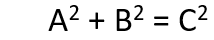You know the values of sides A and B from the resistance and reactance that you plotted, so you can solve this problem for side C by a little algebraic rearranging of the equation to get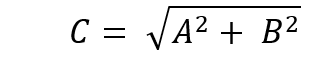Impedance vector magnitude calculation and phase angle calculation.

Plug in the values of A and B to solve asThis means the magnitude of the impedance for the circuit is 500 Ω.

Computing the phase angle is a little headier, requiring some very basic trigonometry. But it’s really quite simple. We’ll use the trigonometry concept of the tangent that relates the angle in question to the two sides of the triangle we plotted as vectors for resistance and reactance. Specifically, we use the inverse of the tangent, or “arctangent.” (This is sometimes depicted on calculators as tan-1.)

First, we compute the value of the tangent of the phase angle as the “opposite side over the adjacent side.” The side opposite the desired angle is the side we plotted as inductive reactance, equal to 300 Ω. The side adjacent to the desired angle (and that is not the hypotenuse side) is the vector we plotted for resistance, equal to 400 Ω. Thus,

Tangent of Phase Angle = 300 / 400 (the fraction: reactance / resistance). Tangent of Phase Angle = 0.75

Now we may determine the angle in degrees by taking the inverse tangent, or arctangent, of 0.75. On most calculators with trigonometric functions this is a “shift” key followed by the “tan” key. (Be sure “degrees” mode is selected, and not “radians.”)

Phase Angle = 37 degrees

We may now state the complex impedance (Z) of the circuit in polar form as 500 Ω at 37 degrees. Since this phase angle is due to inductive reactance we know that the voltage leads the current by 37 degrees. We can also recognize this inductive case because the angle is a positive value. Reflecting back to the voltage-current waveform relationship diagrams we used in Part 1, the 37 degree phase angle appears as follows:Voltage leads the current by 37 degrees, indicated by the phase angle calculation for Z = 400 + j300 Ω

The polar form of impedance states the magnitude in ohms and the phase angle in degrees.

Now let’s consider a capacitive reactance and resistance series circuit case. It is very similar to the inductive reactance case.

Q. What is the impedance of the circuit consisting of a 300-Ω resistor in series with a capacitor that has 400 Ω of reactance?

Again, we construct a vector diagram to help us keep straight the scenario. A 300-Ω resistance is plotted as a vector from the origin along the resistance axis. Then we plot the 400-Ω capacitive reactance beginning at the head of the resistance vector.

But this time we plot the reactance vector downward instead of upward. By standard convention all capacitive reactances are indicated in the –Y direction and with negative phase angles. In rectangular form this scenario is:

Z = 300 – j 400 Ω

Once we connect the resultant vector from the origin to the head of the reactance vector we again have a magnitude and phase angle to compute to convert to polar form.Vector plot of resistance and capacitive reactance, and resultant impedance vector.

The magnitude is calculated with the same method as before, only this time there is a negative value (-400) to include.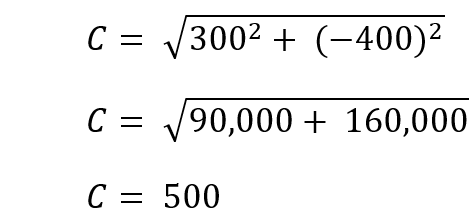Again we have an impedance of magnitude 500 Ω. However, the phase angle will differ…

Tangent of Phase Angle = -400 / 300 Tangent of Phase Angle = -1.333

Again using the arctangent function we find:

Phase Angle = -53 degrees

Thus, Z = 500 Ω at -53 degrees is the polar form definition of this complex impedance. This means that the current leads the voltage by 53 degrees (ICE), and the reactance must be capacitive.

In a rectangular coordinate plane inductive reactance is plotted in the positive Y direction and capacitive reactance is plotted in the negative Y direction, and this is indicated by the sign of the reactance part (j) of the rectangular form notation or the sign of the phase angle in polar form notation.

How about the scenario in which the series circuit contains resistance (R) and both inductive (L) and capacitive (C) reactance, the so-called RLC series circuit? One more example to illustrate the RLC case:

Q. What is the impedance of the circuit consisting of a 4Ω resistor in series with an inductor with 4Ω of reactance and a capacitor with 1Ω of reactance?

We can again start with the rectangular coordinates by plotting the resistance vector of 4Ω.

However, we must compute a single reactance value to plot in the vertical direction. This is where the negation of inductive and capacitive reactances comes into play. You may simply subtract the capacitive reactance from the inductive reactance and plot the result, either positive (up, inductive) or negative (down, capacitive). You may also think graphically of plotting the inductive reactance value up from the head of the resistance vector and then plotting the capacitive reactance value down from the head of the inductive reactance vector.Inductive and capacitive reactances offset one another on the vertical axis.

In this example the result of the offsetting reactances is: X = 4Ω – 1Ω, or X = 3Ω. This is a net inductive reactance (a positive value) and we should complete the vector diagram with a vector to the resultant point of (4, 3). The rectangular form notation here is:

Z = 4 + j 3 Ω

Considering the resultant impedance vector, we may calculate its magnitude and phase angle just as before for the polar form.Arctan (3/4) = 37 degrees (phase angle)

Z = 5Ω at 37 degrees.

With the series circuit impedance under your belt, you’re ready to consider the twists that come with parallel circuits.

Parallel Circuit Impedance: We will tackle a couple of parallel circuit examples, but first we must introduce the concept of admittance. Admittance is the inverse of impedance, and you may think of it as a measure of how easily a circuit will allow current to flow.

Consider a capacitive circuit in which AC of high frequency is readily passed (low impedance) and where AC of low frequency experiences high impedance. The admittance measure for the circuit would be exactly the opposite – the admittance of high frequencies is great in value, and the admittance of low frequencies is small in value.

Admittance is designated with ‘Y’, and it may be computed as Y = 1/Z. The unit of admittance is siemens (S).

Similarly, there are component inverse measures for resistance and reactance, each in units of siemens. The inverse of resistance is called conductivity (G), computed as G = 1/R. The inverse of reactance (X) is called susceptance (B), and B = 1/X.

Admittance (Y) is the inverse of impedance. Y=1/Z Conductivity (G) is the inverse of resistance. G=1/R Susceptance (B) is the inverse of reactance. B=1/X

These inverse measures, called admittances generally, come in handy with parallel circuits. Why? Admittances in parallel add together simply. So, to determine the impedance of a parallel circuit we may perform these steps:

1. Invert the values of resistance and reactance to conductivity and susceptance

3. Revert the summed values back to impedance

Voila! Simple! Well, there is one nuance regarding the phase angle or the rectangular form ‘j’ part. Any time you invert a reactance value the sign of the angle (the sign of the j) flips to the opposite sign. That is, + becomes –, or – becomes + in the course of any inverting or reverting calculations between reactance and susceptance.

Let’s try an example to keep this clear.

Q. What is the impedance of the circuit consisting of a 300-Ω resistor in parallel with an inductor that has 400 Ω of reactance?

G = 1/R [Conductivity is the inverse of resistance] G = 1/300 Ω G = 0.0033 S

AndY = 0.0033 – j 0.0025 S

Third: Compute the admittance(Y) in polar form using the same method as for impedance.Angle Y = Arctan (-0.0025 / 0.0033) Angle Y = -37 deg.

Y = 0.00414 at -37 deg S

Fourth: Revert the admittance (Y) back to impedance.

Z = 1/Y Z = 1/0.00414 at – (-37 deg) [Flip the sign of the angle]

Z = 240 at 37 deg Ω

And there you have it! You can see how the same technique will work for a resistor and capacitor in parallel, only the sign of j or the angle will be the opposite of the parallel inductor example above.

Further, in more elaborate cases where multiple series components are arranged in parallel with other series components, use the series computation method to get a total impedance for each of the series component sets, and then combine series results by the parallel method.

Summary Rules for Computations

2. Inductive reactance and capacitive reactance cancel or offset one another with simple subtraction.

3. Admittance is the inverse, or reciprocal, of impedance. Y=1/Z

5. The sign of j or the sign of the phase angle reverses with each conversion between impedance and admittance.

Impedance Matching Wrap-Up

While we won’t go into great detail on the nuances of impedance matching, you are probably aware that impedance matching is very important for getting the most out of your transmitter-antenna system. Impedance mismatches in a transmission line cause reflections of power that reduce the effective power transfer. You want your transmitter, feed line, and antenna feed point to be closely matched in impedance to avoid high SWR values that indicate the presence of such reflections.

You may recognize now that impedance matching must involve more than just ensuring that the magnitude of impedance is closely matched. Yes, in most transmitters and coaxial cable-based antenna systems we use 50-Ω impedance, and we seek an antenna feed point impedance near that magnitude. But impedance matching also involves the phase angle, or the reactance j part of the impedance. Ideally, we would like the feed point impedance at the antenna to be mostly resistive and have little reactive component to it. That is, impedance with a small or zero value of j is easiest to match. Matching is simpler if there is little or no phase angle difference to wrangle with.

We’ll finish up the discussion with one power transfer principle that may be grasped with the nature of complex impedance now firmly in mind. When the output impedance of a power source (the transmitter) has a significant reactive component (a large j part indicating a phase angle difference), the maximum possible power is delivered to the load (the antenna) if the impedance of the load is equal to the complex conjugate of the power source impedance. What is a complex conjugate, you ask?

Maximum possible power is transferred to a load if the impedances of the source and load are complex conjugates.

A complex conjugate is a pair of numbers, each like the form of the complex impedance rectangular notation, with identical values but opposite signs for the j part. For example:

Z = 53 + j12 Ω

The complex conjugate of this impedance would be

Z = 53 – j12 Ω

Impedance matching devices, such as so-called antenna tuners, may use matching networks of capacitors and inductors to perform complex conjugate impedance matching to aid power transfer. You may have intuited that the complex conjugates provide a matching compensation for phase angle differences, optimizing power transfer for the particular impedance conditions.

Complex impedance is one of the most difficult concepts of radio science to wrap you mind around. I hope our discussion in Parts 1, 2, and 3 have helped you get a better grasp of how complex impedance works and why it is important to electrical and RF systems.

This concludes our series on complex impedance. I hope it helped to clear up some of the mystery and confusion surrounding this topic. Good luck with your studies!

-- Stu WØSTU

2,271 views

See All
bottom of page